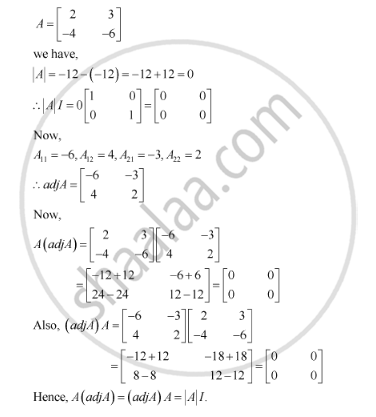Share

# Verify A (Adj A) = (Adj A) A = |A|I [(2,3),(-4,-6)] - CBSE (Commerce) Class 12 - Mathematics

#### Question

[(2,3),(-4,-6)]

#### SolutionIs there an error in this question or solution?

#### Video TutorialsVIEW ALL 

Solution Verify A (Adj A) = (Adj A) A = |A|I [(2,3),(-4,-6)] Concept: Adjoint and Inverse of a Matrix.
S